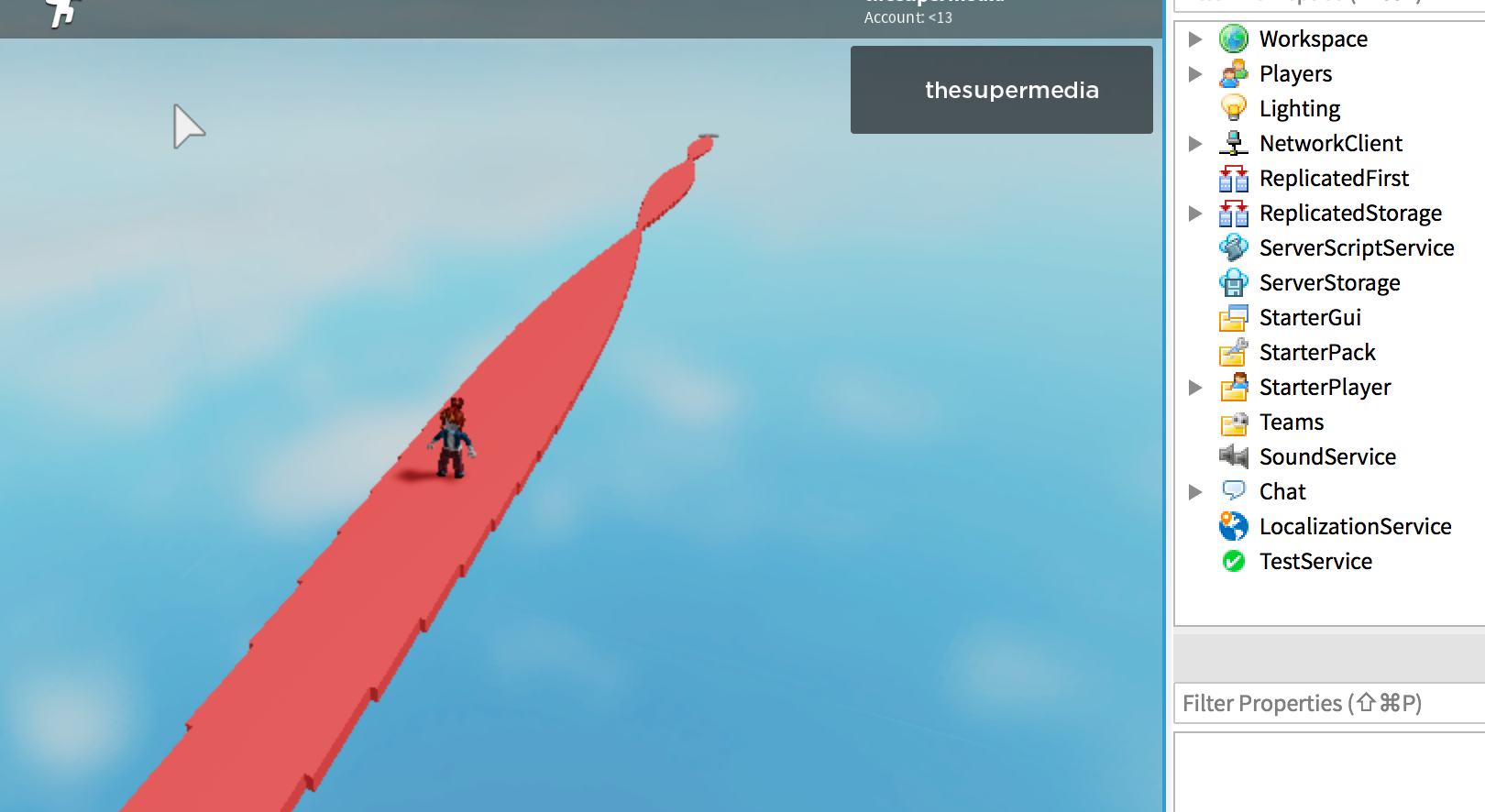Creating a list of tiles (Part) and resize one dimension using math.sin to modulate armonically the shape of the path:The script use a variable `a` that is incremented within the `for-loop`. A new `x` variable is calculated for each tile:

``````local a = 0

for count = 0, 100 do
local p = game.ServerStorage.Tile:Clone()
p.Parent = game.Workspace
p.Position = Vector3.new(0,0,-8.5 - count*5)

local x = math.abs(math.sin(a)*10) + 1
p.Size = Vector3.new(x, 1, 5)
a = a + 0.1
end

``````